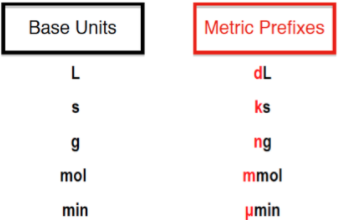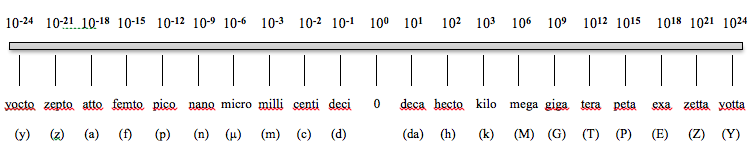## Analytical Chemistry

Learn the toughest concepts covered in your Analytical Chemistry class with step-by-step video tutorials and practice problems.

1. Chemical Measurements

# Metric Prefixes

Metric Prefixes serve as labels for common base units.

Metric Prefix Multipliers
1
concept

## Metric Prefix2m
Play a video:
in this video, let's explore metric prefixes we're gonna say here. Generally we use scientific notation anytime we're trying to change a very large number or very small number into something that's more manageable. But when it comes to analytical chemistry we need to go a step further and traditionally we'll use metric prefixes in order for us to convert very large and very small numbers into something that's easier for us to understand. Now, metric prefixes serve as just a label for common base units. For example. Um one kilometer is equal to 10 to the three m or one micro leader equals 10 to the negative. Six leaders realize here that our metric prefixes are the labels that go in front of some common types of base units. So base unit of meters here and the base units of leaders here We're accustomed to seen when it comes to metric prefixes as being between 10 to the negative 12 To 10 to the 12. This is what we've customarily seen before. But remember analytical chemistry is a chemistry of precision. And because of that we're gonna have to expand the way we look at metric prefixes and look at additional ones that we may not have known beforehand. So this includes expanding all the way to 10 to the negative 24 And all the way up to 10 to the 24. So here we have our basic multiplier for our metric prefixes on top 10 to the negative 24 to 10 to the 24 below. We have the name of that particular metric prefix from yoke toe all the way to Yoda And then the different symbols that are associated with them. Now remember here 10 of the zero serves as just our base In terms of this graph and we're going to say here that remember anytime we do any types of metric prefix conversions we're gonna say that one is associated with our metric prefix. That's why I said that one kg, 1 kg meter is 10 to the three so one kg is 10 to the three. That's why I said that. One micro So micro one micro which is right here is 10 to the negative six up here as we delve deeper and deeper into analytical chemistry. Realize that metric prefixes will always be in play in some way or another. So it's important to commit to memory this chart that we have here in terms of our metric prefix conversions from this will move on to example one. What we put into action some of the key principles we've just learned. So come back, take a look at the next video and see how we approach calculating different types of metric prefixes when it comes to any type of word probWhenever using the metric prefix chart, remember that “1” is associated with the metric prefix.

2
example

## Metric Prefix4m
Play a video: